# 13 Results

View
Selected filters:
• MCCRS.Math.Content.HSA-CED.A.3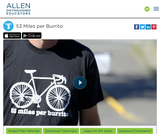Conditions of Use:
Remix and Share
Rating

In this engineering, math, and sustainability project students answer the question, “Can I ride 53 miles on a bike from the energy of a single burrito?” They must define their variables, collect and analyze their data, and present their results. By the end of this project, developed by Allen Distinguished Educator Mike Wierusz, students should have all the information they need to design a burrito that would provide them with the exact caloric content necessary to ride 53 miles.

Subject:
Mathematics
Material Type:
Activity/Lab
Lesson Plan
Provider:
Allen Distinguished Educators
07/27/2016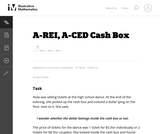Conditions of Use:
No Strings Attached
Rating

The given solutions for this task involve the creation and solving of a system of two equations and two unknowns, with the caveat that the context of the problem implies that we are interested only in non-negative integer solutions. Indeed, in the first solution, we must also restrict our attention to the case that one of the variables is further even.

Subject:
Mathematics
Algebra
Material Type:
Activity/Lab
Provider:
Illustrative Mathematics
Provider Set:
Illustrative Mathematics
Author:
Illustrative Mathematics
01/18/2013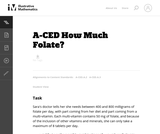Conditions of Use:
No Strings Attached
Rating

This is a task from the Illustrative Mathematics website that is one part of a complete illustration of the standard to which it is aligned. Each task has at least one solution and some commentary that addresses important asects of the task and its potential use. Here are the first few lines of the commentary for this task: Sara's doctor tells her she needs between 400 and 800 milligrams of folate per day, with part coming from her diet and part coming from a multi-vitamin...

Subject:
Mathematics
Material Type:
Activity/Lab
Provider:
Illustrative Mathematics
Provider Set:
Illustrative Mathematics
Author:
Illustrative Mathematics
05/27/2013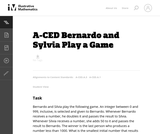Conditions of Use:
No Strings Attached
Rating

This task presents a simple but mathematically interesting game whose solution is a challenging exercise in creating and reasoning with algebraic inequalities. The core of the task involves converting a verbal statement into a mathematical inequality in a context in which the inequality is not obviously presented, and then repeatedly using the inequality to deduce information about the structure of the game.

Subject:
Mathematics
Algebra
Material Type:
Activity/Lab
Provider:
Illustrative Mathematics
Provider Set:
Illustrative Mathematics
Author:
Illustrative Mathematics
10/21/2013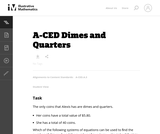Conditions of Use:
No Strings Attached
Rating

This task does not actually require that the student solve the system but that they recognize the pairs of linear equations in two variables that would be used to solve the system. This is an important step in the process of solving systems.

Subject:
Mathematics
Algebra
Material Type:
Activity/Lab
Provider:
Illustrative Mathematics
Provider Set:
Illustrative Mathematics
Author:
Illustrative Mathematics
05/01/2012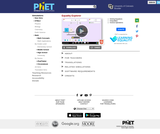Conditions of Use:
No Strings Attached
Rating

Explore what it means for a mathematical statement to be balanced or unbalanced by interacting with objects on a balance. Discover the rules for keeping it balanced. Collect stars by playing the game!

Subject:
Mathematics
Material Type:
Simulation
Provider:
Provider Set:
PhET Interactive Simulations
Author:
David Wood
Marleene Buttice
Meenakshi Raghavan
03/06/2019Rating

This lesson unit is intended to help teachers assess how well students are able to: use the Pythagorean theorem to derive the equation of a circle; and translate between the geometric features of circles and their equations.

Subject:
Geometry
Material Type:
Assessment
Lesson Plan
Provider:
Shell Center for Mathematical Education
U.C. Berkeley
Provider Set:
Mathematics Assessment Project (MAP)
04/26/2013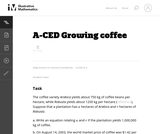Conditions of Use:
No Strings Attached
Rating

This task is designed to make students think about the meaning of the quantities presented in the context and choose which ones are appropriate for the two different constraints presented. In particular, note that the purpose of the task is to have students generate the constraint equations for each part (though the problem statements avoid using this particular terminology), and not to have students solve said equations.

Subject:
Mathematics
Algebra
Material Type:
Activity/Lab
Provider:
Illustrative Mathematics
Provider Set:
Illustrative Mathematics
Author:
Illustrative Mathematics
05/01/2012Rating

This lesson unit is intended to help teachers assess how well students are able to use geometric properties to solve problems. In particular, it will help you identify and help students who have difficulty: decomposing complex shapes into simpler ones in order to solve a problem; bringing together several geometric concepts to solve a problem; and finding the relationship between radii of inscribed and circumscribed circles of right triangles.

Subject:
Geometry
Material Type:
Assessment
Lesson Plan
Provider:
Shell Center for Mathematical Education
U.C. Berkeley
Provider Set:
Mathematics Assessment Project (MAP)
04/26/2013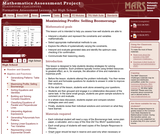Rating

This lesson unit is intended to help teachers assess how well students are able to: interpret a situation and represent the constraints and variables mathematically; select appropriate mathematical methods to use; explore the effects of systematically varying the constraints; interpret and evaluate the data generated and identify the optimum case, checking it for confirmation; and communicate their reasoning clearly.

Subject:
Measurement and Data
Material Type:
Assessment
Lesson Plan
Provider:
Shell Center for Mathematical Education
U.C. Berkeley
Provider Set:
Mathematics Assessment Project (MAP)
04/26/2013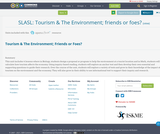Rating

This unit includes 4 lessons where in Biology, students design a proposal or program to help the environment at a tourist location and in Math, students will calculate how tourism affects the economy.

Using inquiry-based reading, students will explore an anchor text and then develop their own essential and supporting questions to guide their research.

Over the course of the unit, students will explore a variety of texts and grow in their knowledge of the impact of tourism on the environment and the economy. They will also grow in their ability to use informational text to support their inquiry and research.

Subject:
Applied Science
Mathematics
Material Type:
Lesson Plan
Author:
Robin Williams
Laura Armstrong
Danielle Gasser
11/06/2017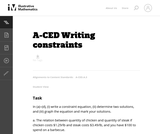Conditions of Use:
No Strings Attached
Rating

The purpose of this task is to give students practice writing a constraint equation for a given context. Instruction accompanying this task should introduce the notion of a constraint equation as an equation governing the possible values of the variables in question.

Subject:
Mathematics
Algebra
Material Type:
Activity/Lab
Provider:
Illustrative Mathematics
Provider Set:
Illustrative Mathematics
Author:
Illustrative Mathematics